# Common multiples and common FactorsPage 1

#### WATCH ALL SLIDES

Slide 1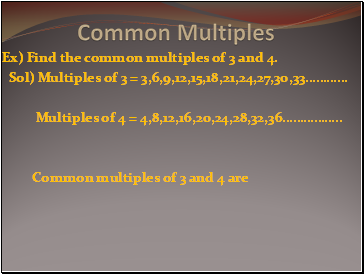## Common Multiples

Ex) Find the common multiples of 3 and 4.

Sol) Multiples of 3 = 3,6,9,12,15,18,21,24,27,30,33…………

Multiples of 4 = 4,8,12,16,20,24,28,32,36……………

Common multiples of 3 and 4 are

Slide 2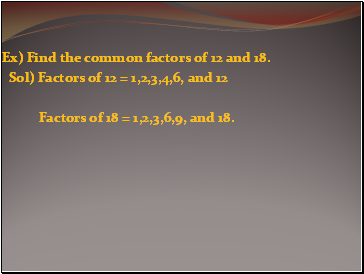Ex) Find the common factors of 12 and 18.

Sol) Factors of 12 = 1,2,3,4,6, and 12

Factors of 18 = 1,2,3,6,9, and 18.

Slide 3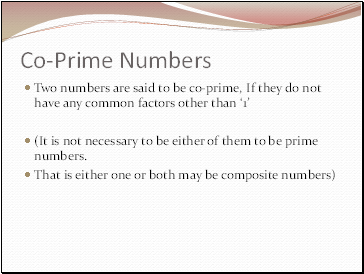## Co-Prime Numbers

Two numbers are said to be co-prime, If they do not have any common factors other than ‘1’

(It is not necessary to be either of them to be prime numbers.

That is either one or both may be composite numbers)

Slide 4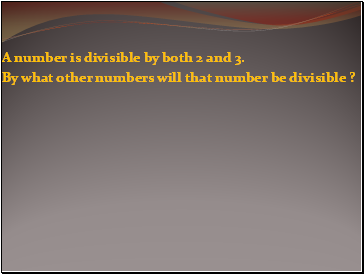A number is divisible by both 2 and 3.

By what other numbers will that number be divisible ?

Slide 5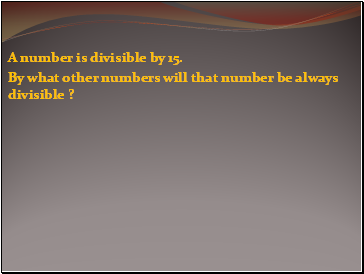A number is divisible by 15.

By what other numbers will that number be always divisible ?